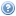# Course List for 2020The undergraduate courses on this list are also available in a timetable view.

Course Title Trimester(s) Teaching Home
COMP 312 Simulation and Stochastic Models 1
ENGR 121 Engineering Mathematics Foundations 1, 2, 3
ENGR 122 Engineering Mathematics with Calculus 2, 3
ENGR 123 Engineering Mathematics with Logic and Statistics 2, 3
MATH 177 Probability and Decision Modelling 2
MATH 243 Multivariable Calculus 2
MATH 245 Computational Mathematics 2
MATH 251 Linear Algebra 2
MATH 277 Mathematical Statistics 1
MATH 311 Algebra 1
MATH 317 Metric Spaces 1
MATH 318 Hilbert Spaces 2
MATH 324 Coding and Cryptography 2
MATH 335 Computability and Complexity 2
MATH 377 Probability and Random Processes 1
MATH 434 Set Theory 1
MATH 435 Computability and Complexity 2
MATH 436 Galois Theory and Number Theory 2
MATH 441 Measure Theory 1
MATH 452 Topology 1
MATH 461 Differential Equations 2
MATH 477 Probability 1
MATH 482 Special Topic: Infinite Combinatorics 1
MATH 483 Special Topic: Operator Algebra 1
MATH 488 Research Project 2 1, 2
STAT 193 Statistics in Practice 1, 2, 3
STAT 293 Applied Statistics 2B 2
STAT 332 Statistical Inference 2
STAT 393 Linear Models 2
STAT 394 Multivariate Statistics 2
STAT 432 Computational Statistics 2
STAT 433 Stochastic Processes 2
STAT 434 Statistical Inference 2
STAT 438 Generalised Linear Models 1
STAT 440 Directed Individual Study 1, 3
STAT 441 Directed Individual Study 1, 2